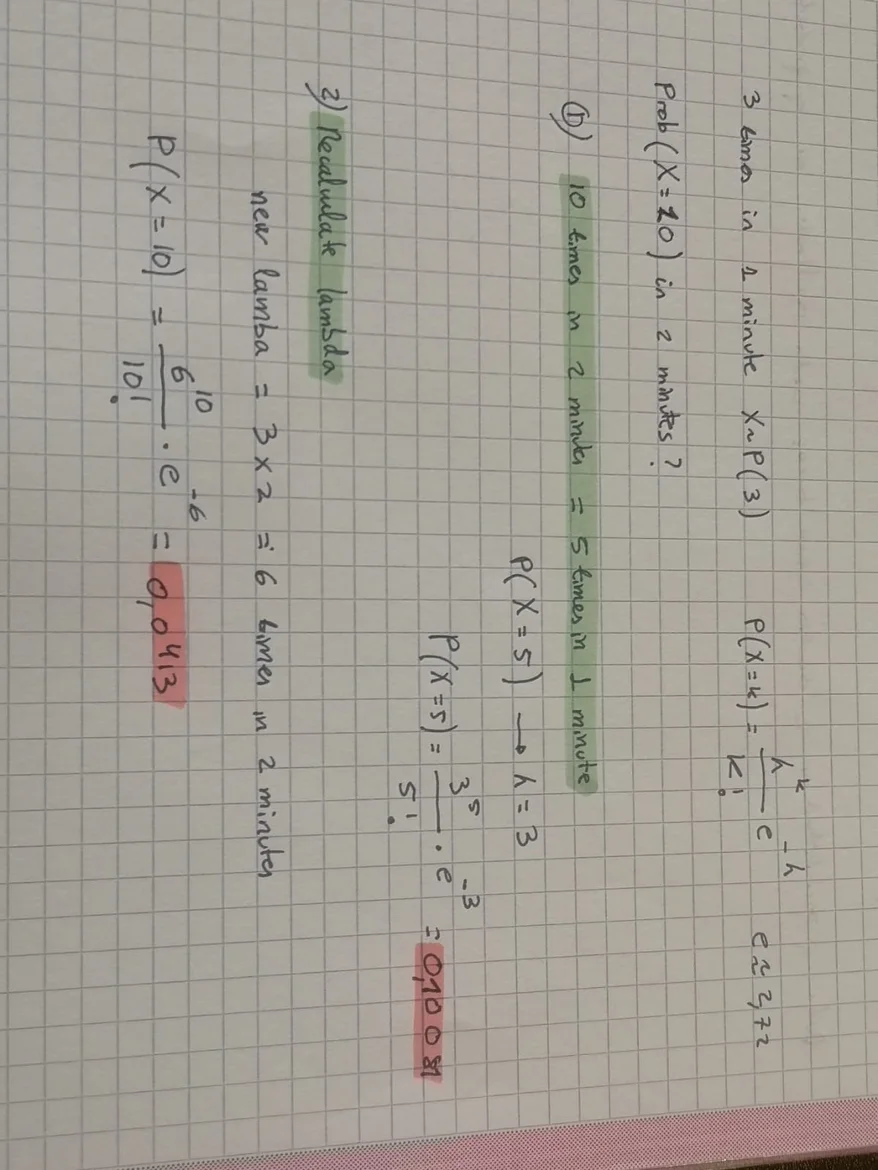30 Oct 2023

Posted on:

28 Oct 2023

0

# Resolved:Doubt Poisson Distribution

Good morning. Thinking about the example of the firefly that lights up 3 times per minute, this means that it follows a Poisson with lambda = 3 for a time of 1 minute, if you wanted to calculate the probability that it lights up 10 times in 2 minutes.
Can I do 10 times in 2 minutes is same as 5 times in 1 minute and use Poisson of lambda = 3 ? Or do I have to recalculate lambda and therefore a new Poisson which would be with lambda =2 (3 ignitions per minute * 2)

And then calculate the probability

I ask because the probabilities using one or another method give me very different, I have searched on the web and I have seen that lambda is recalculated, but in some places it is said that it would not be necessary due to the principle of the constant rate of occurrence.
But as you can see in the photo, the probabilities are very different, I hope you can solve my doubt.
Thanks in advance :)Instructor
Posted on:

30 Oct 2023

0

Hi Enrique,

If a firefly lights up 3 times per minute, the rate parameter λ for 1 minute is 3. If you're looking at a 2-minute window, the total expected number of times the firefly lights up doubles. So, for a 2-minute window, λ=3×2=6.

To calculate the probability that the firefly lights up 10 times in a 2-minute period, you would use:

P(X=10) for λ =6

P(X=10) = 6^10 * e^ (-6) / (10!) - like you've done in the second formula!

It is incorrect to consider 10 times in 2 minutes as being the same as 5 times in 1 minute. The correct approach is to recalculate λ for the 2-minute interval, which would be 6, and then use that in the Poisson formula.

Hope this helps!

Best,

Ned

Posted on:

30 Oct 2023

0

thx ;)